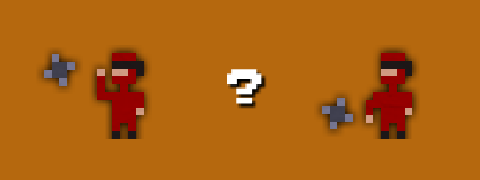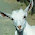## Monday, April 11, 2011

### Randomization In Games - Managing Player ExpectationsGenerating random numbers for use in games is easy. In C#, it is as simple as creating an instance of the Random class and calling Next() on it. Want to decide if an event should occur given a particular probability? Easy, just call Random.Next() and check if the result is less that your probability (expressed as a value from 0 to 1).

The results of this simple approach, however, do not always do a good job of creating behavior that corresponds to a player's expectations. This is because the results are truly random (ok, psuedorandom - but that's generally random enough) and events are independent. By independent, I mean that the result of a probability check does not depend in any interesting way on previous checks. Like flipping a coin, it doesn't matter if you have gotten 9 heads in a row - the probability of getting heads on the next flip is still exactly 50%.

People, however, have a tendency to assume that past result *do* influence future ones. In the case of flipping a coin, this assumption is irrational. In other cases, though, it isn't so much. A key example of this is selecting items randomly from a collection. If you replace items between choosing, then each selection is random and independent of the others. If, however, you do not replace items that you have chosen, this is no longer the case.

The notion of selecting items from a collection without replacement is a very useful one in games, and can result in random behavior that is much more in line with player expectations than independent probability checks.

In working on Kung Fu FIGHT!, I ran into this problem a lot. For example, when an enemy throws a shuriken, I have to decide if they should throw high or low. I started with a simple 50% probability check, but this sometimes resulted in a long string of high or low throws in a row. Perfectly reasonable from a probability perspective, but not what I wanted. On the other hand, simply alternating between high and low seemed too regular.

What to do?

I created a simple helper class to allow me to select random values from a finite "bucket". Here is how it works for my shuriken throwing behavior. To initialize the bucket:

```Random random = new Random();

```

What this does is initialize a bucket and fill it with two 'trues' and two 'falses'.

Then, to decide to throw high or low, simply do:

```if (throwBucket.Choose())
{
// throw high
}
else
{
// throw low
}
```

Each time we call Choose(), a random value is selected from the bucket and take out of the list of available values. When we run out of values, the bucket is refilled with its original contents.

The result of this is random behavior with local dependencies. Each time I select a value, the probability that I get that value again next time goes down. You can't get more than four trues or falses in a row - and even that is fairly unlikely. If we only put 1 true and one false in the bucket instead of two of each, the behavior becomes even more locally dependent. Adding more values in makes things more random.

In addition to having nice properties for game randomness, the RandomBucket class also make for simple, clean code. For example, when I shoot out spark particles for bombs, I use a color bucket to choose what color a spark is:

```RandomBucket<Color> colors =

Color sparkColor = colors.Choose();```

Here I am spitting out the colors in equal volumes, but I could easily change the ratios by altering the numbers when seeding the bucket.

Here is the RandomBucket class. As you can see, it is pretty simple. It doesn't generate garbage (an important quality for Xbox development) and it is quite efficient for reasonably small numbers of values, which should be the case since if you use a large number of values you might as well just use true randomness.

```using System;
using System.Collections.Generic;

namespace MyCoolNamespace
{
public class RandomBucket<T>
{
List<T> chooseList = new List<T>();
Random random;

public RandomBucket(Random random)
{
this.random = random;
}

public RandomBucket<T> Add(T value, int number)
{
for (int i = 0; i < number; i++)
{
}

return this;
}

public T Choose()
{
if (chooseList.Count == 0)
{
List<T> tmp = chooseList;
}

int pos = random.Next(chooseList.Count);

T obj = chooseList[pos];

chooseList.RemoveAt(pos);

return obj;
}

public void Clear()
{
chooseList.Clear();
}
}
}
```

1.Nice post. I never really thought about randomness this way but I can definitely see how this would better meet player expectations.

PS: Nice clean code too!

2.That's cool. Very simple and effective. Damn users and their damn expectations! :)

3.This is very similar to the way that Tetris selects its pieces also.

4.Instead of discarding to the discardList, you could have newList that contains the original items, then instead of discarding to the list and swapping when you are done, you could say chooseList = newList. This would give you exactly the same effect.

5.Hi Chris - actually, that wouldn't work, at least not as you have described. "chooseList" and "newList" are just pointers.

I suppose you could have a separate list of the original items and copy all of its elements to "chooseList", but that seems more complicated and wouldn't be any more efficient.

6.Hi Mike,

Do you mind if I borrow this code for a project I'm working on? I know it's pretty simple but just thought I'd be polite and ask rather than re-write it. I can credit you in the code of course.

7.Absolutely - I posted it because I thought others might find it useful.

8.Awesome. Thanks.